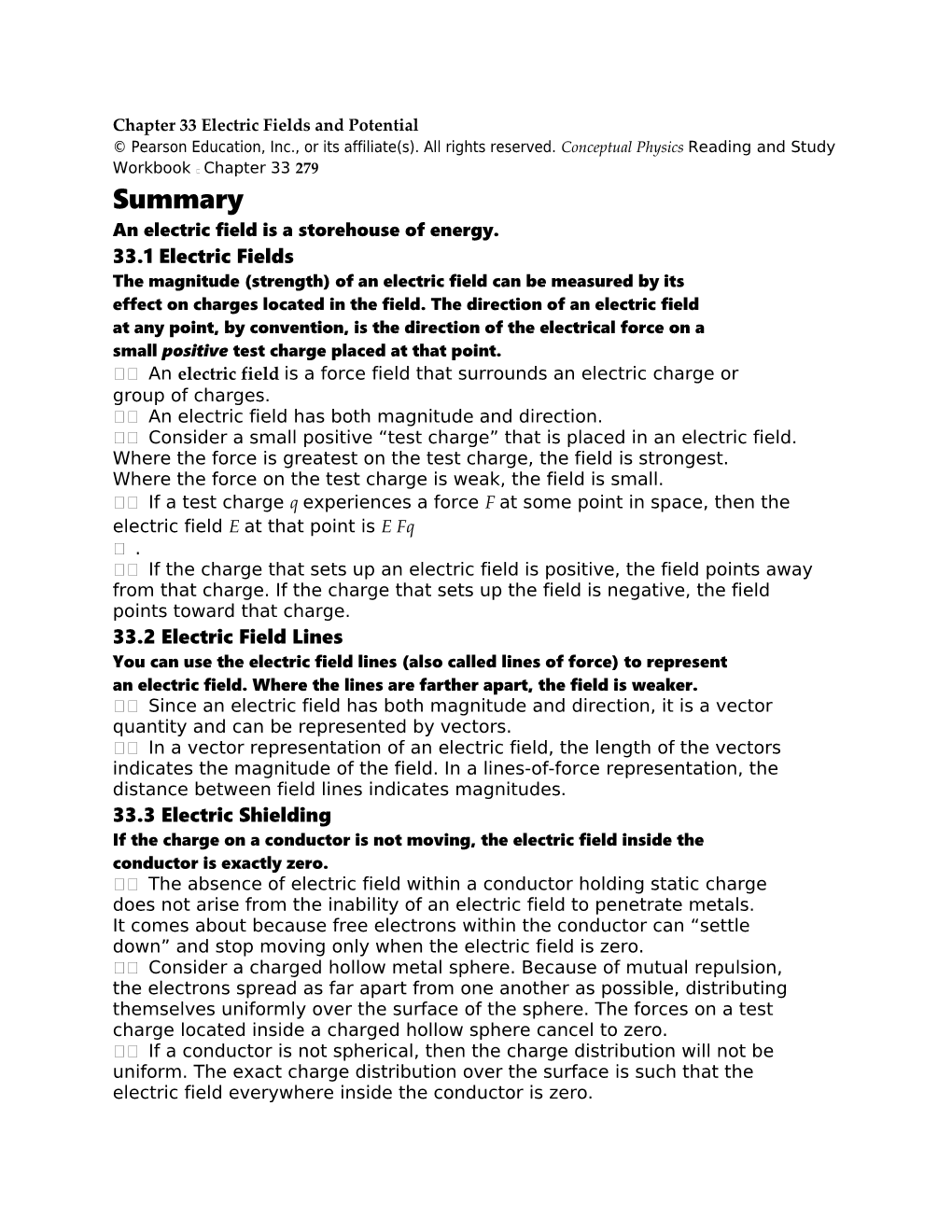# Chapter 33 Electric Fields and PotentialChapter 33 Electric Fields and Potential

Summary

An electric field is a storehouse of energy.

33.1 Electric Fields

The magnitude (strength) of an electric field can be measured by its

effect on charges located in the field. The direction of an electric field

at any point, by convention, is the direction of the electrical force on a

small positive test charge placed at that point.

??An electric field is a force field that surrounds an electric charge or

group of charges.

??An electric field has both magnitude and direction.

??Consider a small positive “test charge” that is placed in an electric field.

Where the force is greatest on the test charge, the field is strongest.

Where the force on the test charge is weak, the field is small.

??If a test charge q experiences a force F at some point in space, then the

electric field E at that point is E Fq

?.

??If the charge that sets up an electric field is positive, the field points away

from that charge. If the charge that sets up the field is negative, the field

points toward that charge.

33.2 Electric Field Lines

You can use the electric field lines (also called lines of force) to represent

an electric field. Where the lines are farther apart, the field is weaker.

??Since an electric field has both magnitude and direction, it is a vector

quantity and can be represented by vectors.

??In a vector representation of an electric field, the length of the vectors

indicates the magnitude of the field. In a lines-of-force representation, the

distance between field lines indicates magnitudes.

33.3 Electric Shielding

If the charge on a conductor is not moving, the electric field inside the

conductor is exactly zero.

??The absence of electric field within a conductor holding static charge

does not arise from the inability of an electric field to penetrate metals.

It comes about because free electrons within the conductor can “settle

down” and stop moving only when the electric field is zero.

??Consider a charged hollow metal sphere. Because of mutual repulsion,

the electrons spread as far apart from one another as possible, distributing

themselves uniformly over the surface of the sphere. The forces on a test

charge located inside a charged hollow sphere cancel to zero.

??If a conductor is not spherical, then the charge distribution will not be

uniform. The exact charge distribution over the surface is such that the

electric field everywhere inside the conductor is zero.

33.4 Electrical Potential Energy

The electrical potential energy of a charged particle is increased when

work is done to push it against the electric field or something else that

is charged.

??A charged object can have potential energy by virtue of its location in an

electric field.

??Suppose you have a small positive charge located at some distance from

a positively charged sphere. If you push the small charge closer to the

sphere, you expend energy to overcome electrical repulsion. The work is

equal to the energy gained by the charge.

??The energy a charge has due to its location in an electric field is called

electrical potential energy.

33.5 Electric Potential

Electric potential is not the same as electrical potential energy. Electric

potential is electrical potential energy per charge.

??The concept of electrical potential energy per charge has a special name,

electric potential: electric potential = electrical potential energy/charge.

??The SI unit of measurement for electric potential is the volt.

??Since potential energy is measured in joules and charge is measured in

coulombs, 1 volt = 1 joule/coulomb.

??Since electric potential is measured in volts, it is commonly called

voltage.

33.6 Electrical Energy Storage

The energy stored in a capacitor comes from the work done to

charge it.

??Electrical energy can be stored in a common device called a capacitor.

??The simplest capacitor is a pair of conducting plates separated by a small

distance, but not touching each other. When the plates are connected to

a charging device such as a battery, charge is transferred from one plate

to the other. The greater the battery voltage and the larger and closer the

plates, the greater the charge that is stored.

33.7 The Van de Graaff Generator

The voltage of a Van de Graaff generator can be increased by increasing

the radius of the sphere or by placing the entire system in a container

filled with high-pressure gas.

??In a Van de Graaff generator, a moving rubber belt carries electrons from

the voltage source to a conducting sphere.

??Van de Graaff generators in pressurized gas can produce voltages as high

as 20 million volts. These devices accelerate charged particles used as

projectiles for penetrating the nuclei of atoms.